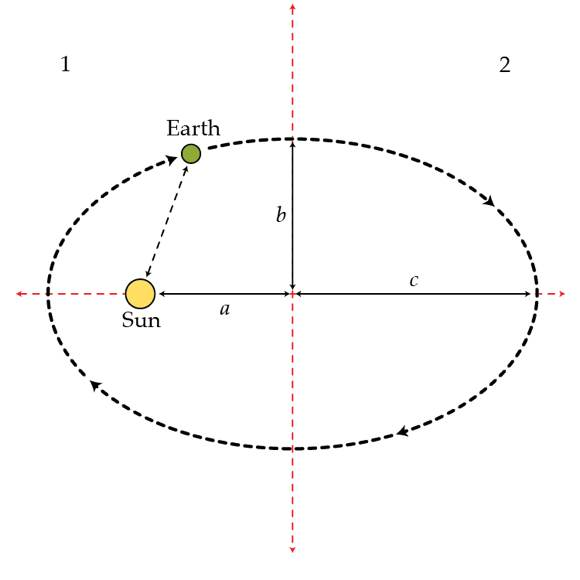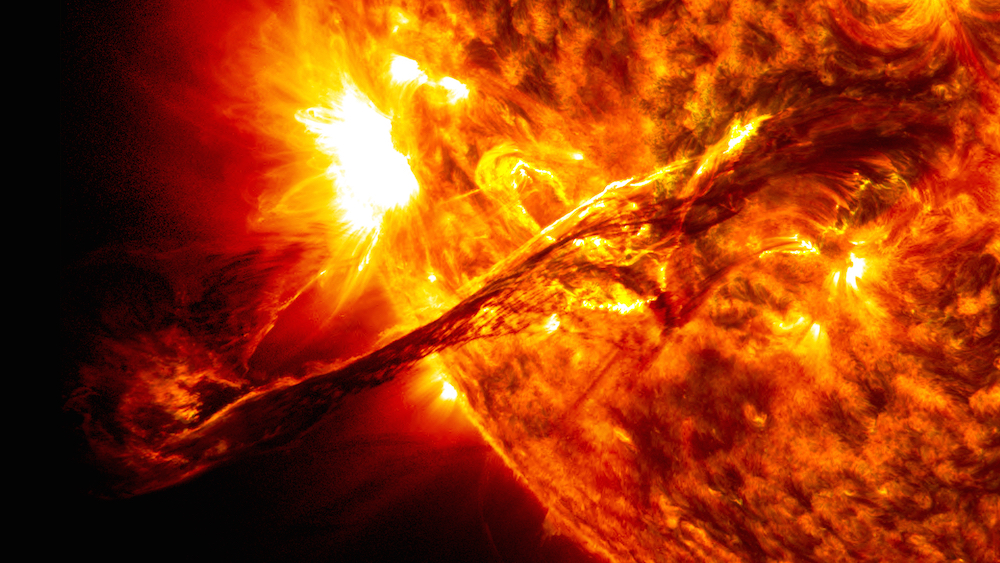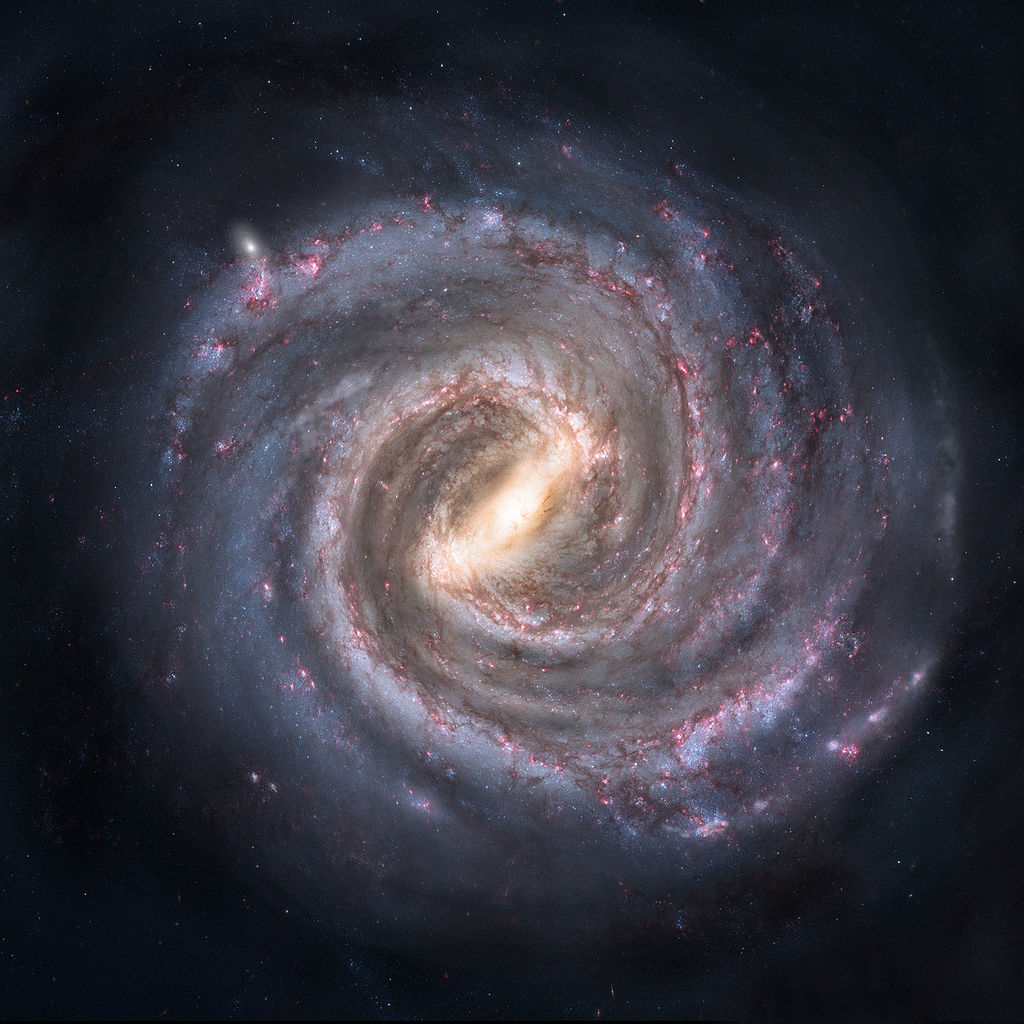Classical Mechanics

# Orbits: Level 2-4 Challenges

A satellite is in a circular orbit very close to the surface of a planet of uniform density $\rho$. What is the period (in hours) of the satellite's orbit around the planet?

Details and Assumptions

• $G = 6.67 \times {10}^{-11} N\cdot{m}^{2}/{kg}^{2}$
• $\rho = 4.5 \frac{g}{{cm}^{3}}$

Astronomers determine masses of distant objects by observing their smaller companions. For example, they can measure the period of a planet orbiting around a distant star, measure the radius of its circular orbit, and then employ Newtonian physics to determine the mass of the star.

Callisto is one of the Jupiter's moons. Its period of revolution is $16.7~\mbox{days}$, and the radius of its circular trajectory is $1.9 \times 10^6~\mbox{km}$. What is Jupiter's mass in kg?

Details and assumptions

• The mass of Callisto is much smaller than the mass of Jupiter.

Suppose the Earth's orbit is divided in two by its minor axis as shown in the figure. How much longer in days does the Earth spend in one half than the other? The eccentricity of an elliptical orbit is defined as $e:=\frac{a}{c}$ where $c$ is the semi-major axis and $a$ is the distance from the the center of ellipse to a focal point. For the Earth's orbit this number is $e=0.0167.$The Earth orbits around the Sun because it has angular momentum. If we stopped the Earth in orbit and then let it fall straight towards the Sun, how long would it take to reach the sun in seconds?

Details and assumptions

• The mass of the Sun is $2 \times 10^{30}~\mbox{kg}$.
• The mass of the Earth is $6 \times 10^{24}~\mbox{kg}$.
• Newton's constant is $6.67 \times 10^{-11}~\mbox{Nm}^2/\mbox{kg}^2$.
• The Earth is $149,600,000~\mbox{km}$ from the Sun.
• You may treat the Earth and Sun as point masses.Are there any photographs of the Milky Way from this viewpoint?

×